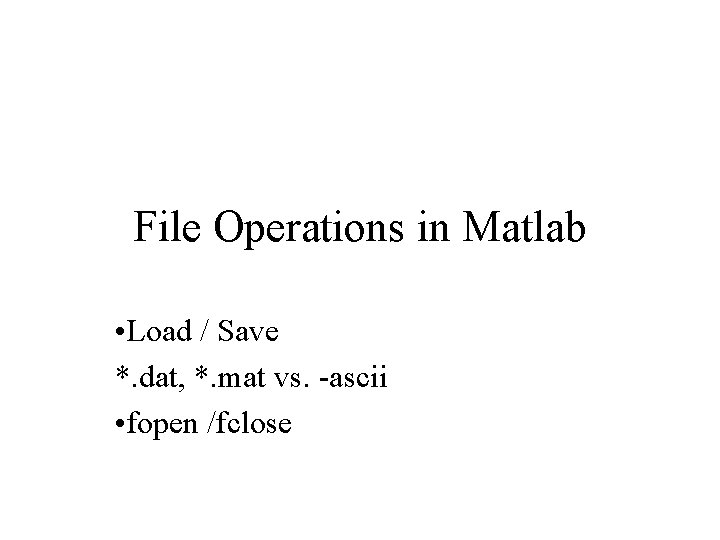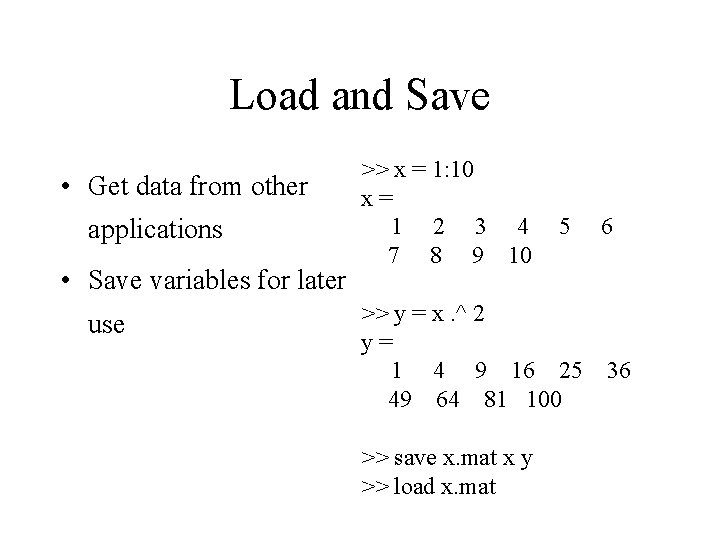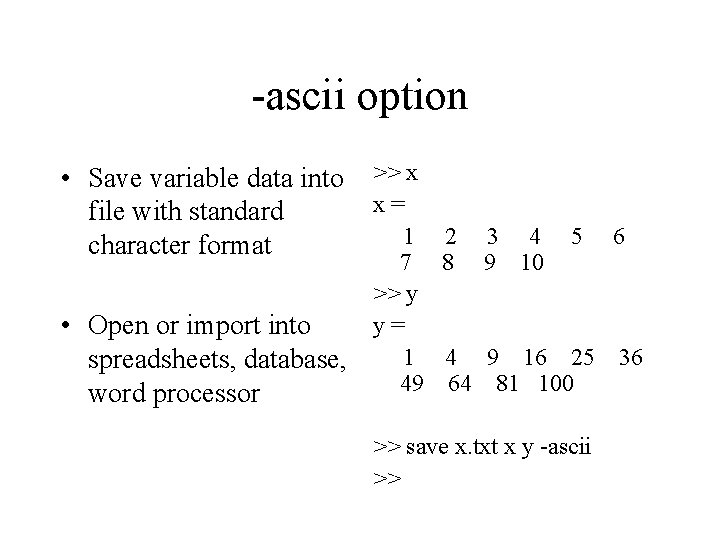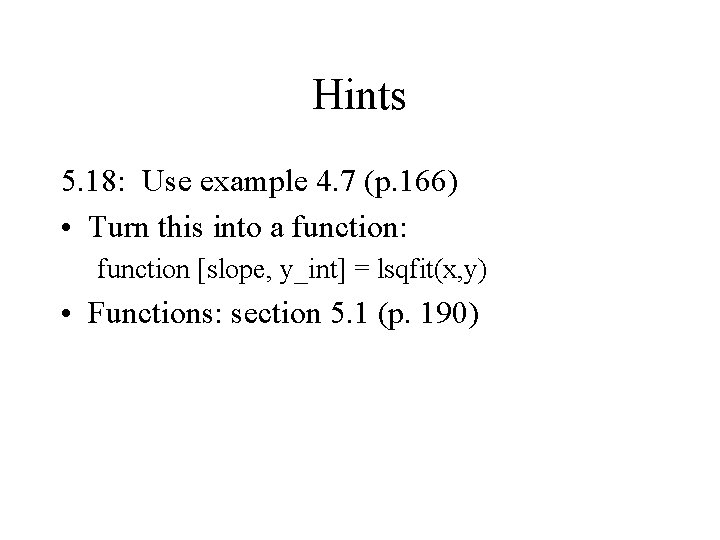# File Operations in Matlab Load Save dat mat

• Slides: 8File Operations in Matlab • Load / Save *. dat, *. mat vs. -ascii • fopen /fcloseLoad and Save • Get data from other applications • Save variables for later use >> x = 1: 10 x= 1 2 3 4 7 8 9 10 5 >> y = x. ^ 2 y= 1 4 9 16 25 49 64 81 100 >> save x. mat x y >> load x. mat 6 36-ascii option • Save variable data into file with standard character format • Open or import into spreadsheets, database, word processor >> x x= 1 2 3 4 5 6 7 8 9 10 >> y y= 1 4 9 16 25 36 49 64 81 100 >> save x. txt x y -ascii >>fopen / fclose • Open files and write or read data into them. • Use the file ID # to specfiy placement of data. • fprintf writes formatted data to files >> fid = fopen('x. mat', 'w+'); >> load x. txt >> fprintf(3, ' %6. 4 fn ', x); >> fid = 3 >> fclose(fid); >>results x. txt x. matHints 5. 18: Use example 4. 7 (p. 166) • Turn this into a function: function [slope, y_int] = lsqfit(x, y) • Functions: section 5. 1 (p. 190)Hints • 5. 22 – Use rand function to generate random number. – Scale from [0, 1) interval to [-1, 1) – Make user-defined function to transform uniform to normal – use this function in m-file to generate array – use hist() function, and std() built in functionsHints • 7. 6 [structure arrays] – – 1. 2. • Define structure array in m-file Create user defined function that accepts structure array plots the info in the array section 7. 3. 4 -5 (p. 310 -311)